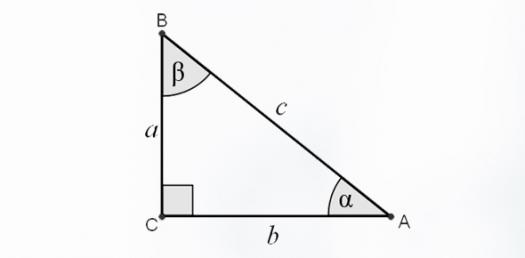Maths Special Right Triangles Quiz 2

5 Questions | Total Attempts: 279SettingsLet's start this quiz it will give you the different right triangles, Find out now how much do you know about it!

• 1.
If you know one side of a 45-45-90 triangle, then you can ALWAYS find the exact length of the other 2 sides.
• A.

True

• B.

False

• 2.
Find x and y.
• A.

X = 5*sqrt(2), and y = 5*sqrt(2)

• B.

X = 5*sqrt(3), and y = 5

• C.

X = 5, and y = 5*sqrt(2)

• D.

X = 5, and y = 5*sqrt(3)

• 3.
No matter what positive number x is, x*sqrt(3) is LARGER than 2x.
• A.

True

• B.

False

• C.

Depends on what positive number x is.

• 4.
Find x and y.
• A.

X=70*sqrt(2), and y=70

• B.

X=140, and y=70*sqrt(3)

• C.

X=70*sqrt(3), and y=140

• 5.
Find x and y.
• A.

X = 15*sqrt(3), and y = 30

• B.

X = 7.5*sqrt(3), and y = 15*sqrt(3)

• C.

X = 5*sqrt(3), and y = 10*sqrt(3)

Related TopicsBack to top